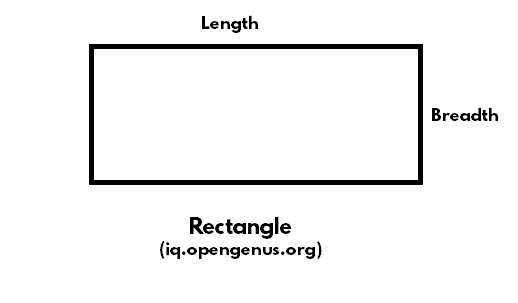×

Search anything:

# Find Perimeter and Area of rectangle in C Programming

#### C ProgrammingIn this article at OpenGenus, we have explained how to find the perimeter and area of a rectangle by using length and breadth (as user inputs). We have demonstrated this with a C implementation.

## Table of content

1. Question
2. Approach to solution
3. Implementation in C
4. Output

## Question

The problem is to find the area and perimeter of a rectangle using C Programming with length and breadth as user input.

Area is defined as the region within a given shape. Perimeter is defined as the total length of the boundary of the given shape.

A rectangle is a 4 sided shape such that two opposite sides always have the same length. It looks as follows:Figure: Rectangle (a 4 sided figure)

For example, if length of a rectange is 10 and breadth is 7.

Then, Area = length * breadth = 70 cm^2
Perimeter = 2 * (length + breadth) = 34 cm

## Approach to solution

1. Library and function

To enable the program to take user input, we need to use stdio.h library in C. stdio stands for "Standard Input and Output". It is used within the main function ("int main()") and is included as follows:

``````#include <stdio.h>
``````
1. Declaration of data type

To specify or inform the computer about what type of data type we are going to use we will use data type. In this type of question, we can use:

• float(float): for showing decimal-type numbers
• double(double): for decimal type numbers
• integers(int): for integer type numbers

Here we will use an integer(int)

1. Introduction to variables

Here we have to use the variables to store the data. The variable used here are:
Length=l
Area=ar
Perimeter=peri

1. The user input format

From the above question, we got to know that we will put the length and breadth as user input so for this we will use:

``````printf("Enter the value of length and breadth of the rectangle :\n");
scanf("%d",&l);
scanf("%d",&b);
``````

Where scanf takes the input

1. To find the area and perimeter of the rectangle

To find the value of area and perimeter, first we have to write the formula of area and perimeter and then print the value of it:

``````area=l*b;
perimeter=2*(l+b);
printf("The value of area is %d\n",ar);
printf("The value of perimeter of rectangle is %d\n",peri);
``````
1. Closing statment

At last, we are going to end the coding of the return 0 command. 0 denotes that the program has executed successfully.

## Implementation in C

Following is the complete C implementation to calculate the area and perimeter of a rectangle:

``````// Part of iq.opengenus.org
#include<stdio.h>
int main()
{
int L, B, AR, peri;
printf("enter the value of length and breadth of the rectangle :\n");
scanf("%d",&L);
scanf("%d",&B);
AR=L*B;
peri=2*(L+B);
printf("the value of area is %d\n",AR);
printf("the value of perimeter of rectangle is %d\n",peri);
return 0;
}
``````

### Output

``````enter the value of the length and breadth of the rectangle :
2
4
the value of the area is 8
The value of the perimeter of the rectangle is 12
``````

With this article at OpenGenus, you must have the complete idea of how to calculate area and perimeter of a rectangle in C Programming Language.#### Akshata Wagji

Akshata Wagji is a 2nd year Computer Science student at Kalinga Institute of Technology (KIIT), Bhubaneswar.

Find Perimeter and Area of rectangle in C Programming